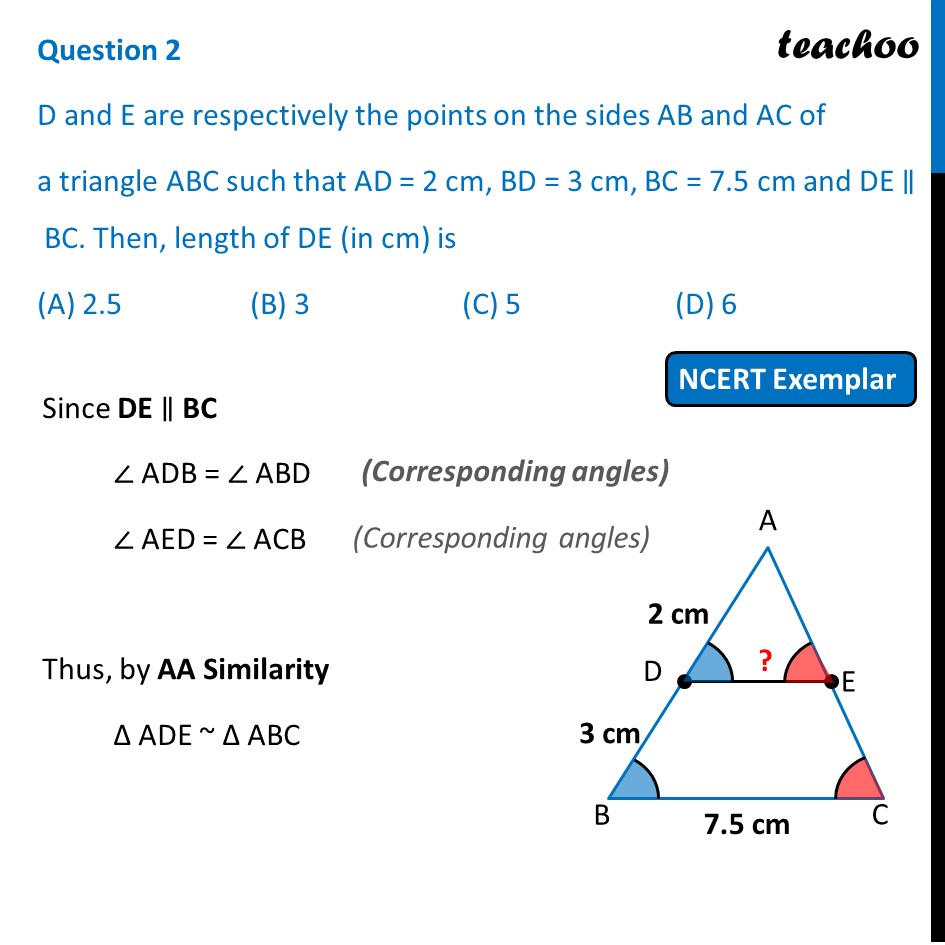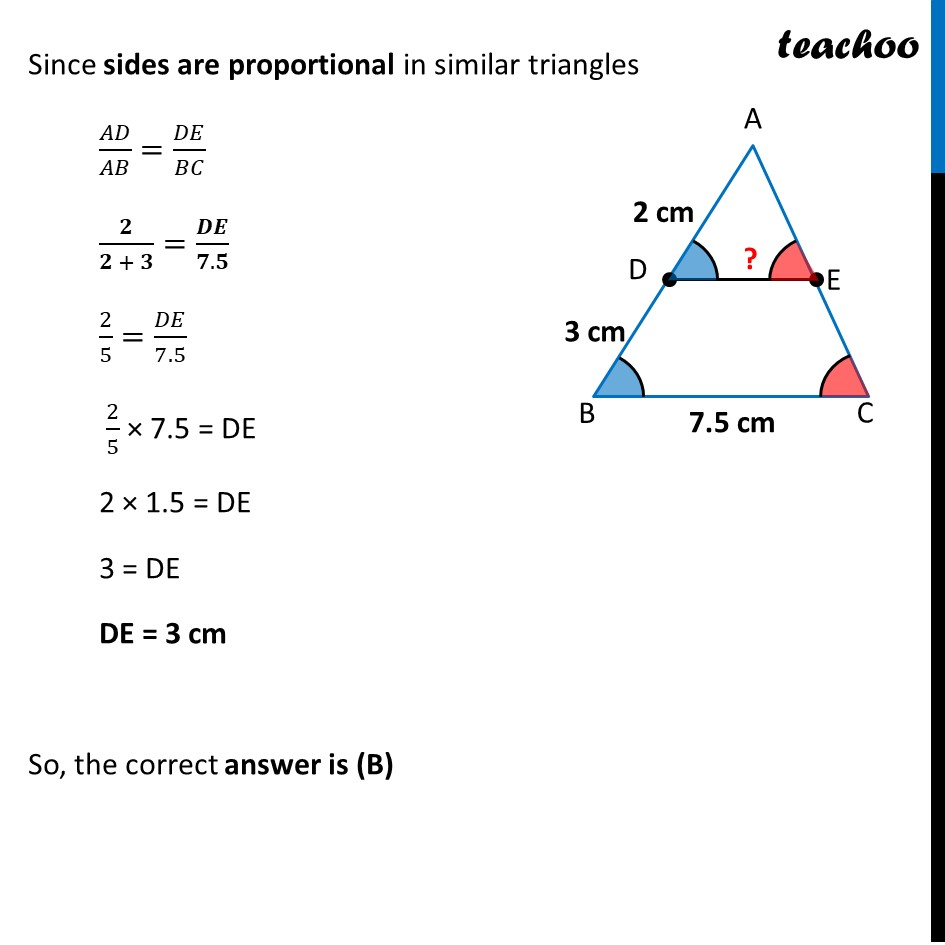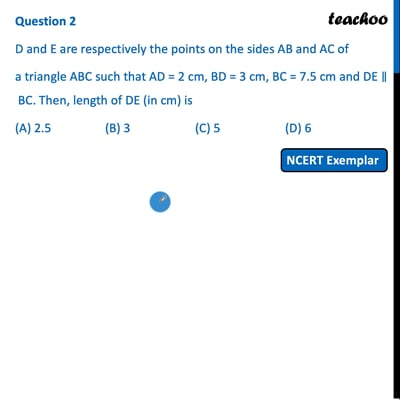NCERT Exemplar - MCQ

Chapter 6 Class 10 Triangles (Term 1)
Serial order wise

## (D) 6This video is only available for Teachoo black users

### Transcript

Question 2 D and E are respectively the points on the sides AB and AC of a triangle ABC such that AD = 2 cm, BD = 3 cm, BC = 7.5 cm and DE ∥ BC. Then, length of DE (in cm) is (A) 2.5 (B) 3 (C) 5 (D) 6 Since DE ∥ BC ∠ ADB = ∠ ABD ∠ AED = ∠ ACB Thus, by AA Similarity Δ ADE ~ Δ ABC (Corresponding angles) (Corresponding angles) Since sides are proportional in similar triangles 𝐴𝐷/𝐴𝐵=𝐷𝐸/𝐵𝐶 𝟐/(𝟐 + 𝟑)=𝑫𝑬/(𝟕.𝟓) 2/5=𝐷𝐸/7.5 2/5 × 7.5 = DE 2 × 1.5 = DE 3 = DE DE = 3 cm So, the correct answer is (B)